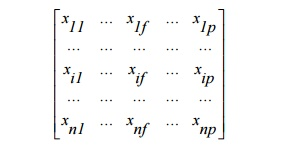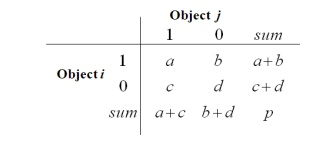Home | | Data Ware Housing and Data Mining | Type of Data in Clustering Analysis

# Type of Data in Clustering Analysis

Data structure Data matrix (two modes) object by variable Structure

TYPE OF DATA IN CLUSTERING ANALYSIS

Data structure Data matrix (two modes) object by variable StructureDissimilarity matrix (one mode) object –by-object structureWe describe how object dissimilarity can be computed for object by Interval-scaled variables,

Binary variables, Nominal, ordinal, and ratio variables, Variables of mixed types

Interval-Scaled variables (continuous measurement of a roughly linear scale) Standardize dataUsing mean absolute deviation is more robust than using standard deviation

Similarity and Dissimilarity Between Objects

Distances are normally used to measure the similarity or dissimilarity between two data objects

Some popular ones include: Minkowski distance:Also, one can use weighted distance, parametric Pearson product moment correlation, or other dissimilarity measures

Binary Variables

A contingency table for binary dataDistance measure for symmetric binary variables:Distance measure for asymmetric binary variables:Jaccard coefficient (similarity measure for asymmetric binary variables):Categorical variables

A generalization of the binary variable in that it can take more than 2 states, e.g., red, yellow, blue, green

Method 1: Simple matching

m: # of matches, p: total # of variablesMethod 2: use a large number of binary variables

creating a new binary variable for each of the M nominal states

Ordinal Variables

An ordinal variable can be discrete or continuous

Order is important, e.g., rank

Can be treated like interval-scaled

replace xif  by their rank

map the range of each variable onto [0, 1] by replacing i-th object in the f-th variablecompute the dissimilarity using methods for interval-scaled variables

Ratio-scaled variable:

a positive measurement on a nonlinear scale, approximately at exponential scale, such as AeBt or

Ae-Bt

Methods:

treat them like interval-scaled variables—not a good choice! (why?—the scale can be distorted)

apply logarithmic transformation yif = log(xif)

treat them as continuous ordinal data treat their rank as interval-scaled

Variables of Mixed Types

A database may contain all the six types of variables symmetric binary, asymmetric binary,

nominal, ordinal, interval and ratio

One may use a weighted formula to combine their effectsVector Objects

Vector objects: keywords in documents, gene features in micro-arrays, etc.

Broad applications: information retrieval, biologic taxonomy, etc.

Cosine measureStudy Material, Lecturing Notes, Assignment, Reference, Wiki description explanation, brief detail
Data Warehousing and Data Mining : Clustering and Applications and Trends in Data Mining : Type of Data in Clustering Analysis |

Related Topics